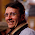## Monday, November 8, 2010

### Find the min difference among two numbers in an Array

Given an array of n integers A, find the minimum difference between two numbers in A. It's easy to make it in O(n log n). Can you make it in O(n)?

1.this is difficult

2.1. Use partiting step from quick sort
2. compare [L/2 + 1] - [L/2] or [L/2] - [L/2 - 1]

3.This does seem hard and I can't figure a way to attack it without that extra logN. It can be solved in order N EXPECTED time but not true O(N) limits. (Interesting: the tougher 2D generalization of the problem, finding 2 points that are closest in a 2D plane, is O(n Log n) but also has an expected order N randomized algorithm. See for example the Kleinburg/Tardos textbook.)

4.vstepanov, that requires you to descend recursively into both halves of the partition, making it O(n log n).

5.Got it!

These are integers so it's possible to use a O(n) radix sort! So sort the numbers, then scan down the list and look at the pairwise differences, return the largest.

If the numbers are reals, I think O(n log n) is the true limit. But computer integers or 32/64 bit floats are sortable in O(n) so this will work.

Good puzzle, I had given up. But that "integer" clue in the problem description was key.

6.Can we make any assumptions about the numbers in the array? like possible range of values?

7.Can you make any assumptions about the integer range?